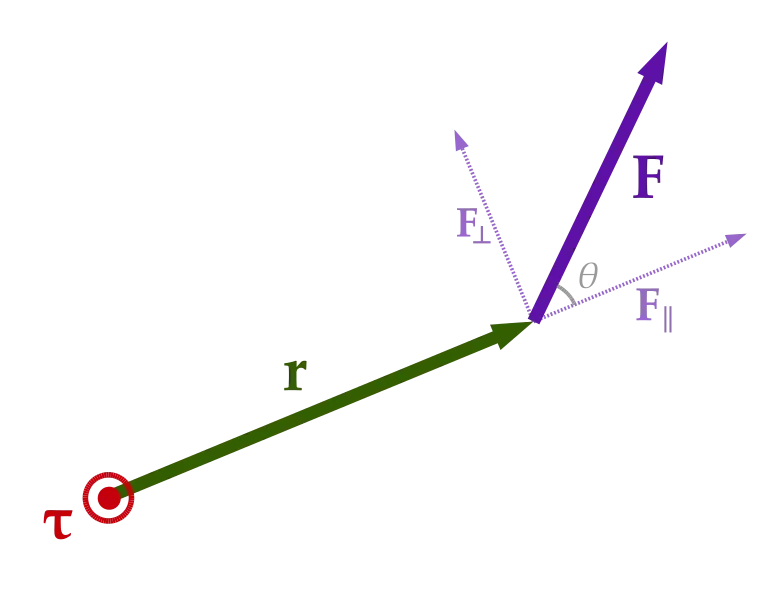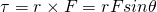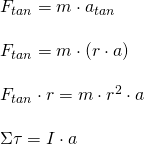# Physics - Classical Mechanics - Torque and Angular Acceleration[Image 1]

## Introduction

Hey it's a me again @drifter1! Today we continue with Physics and more specifically the branch "Classical Mechanics" to get into the quantity called Torque, that's strongly related to Angular Acceleration. So, without further ado, let's get straight into it!

## Torque

Similar to how we define Force to be the measure of how much we have to push an object to make it move from one point to another, we also define a quantity for measuring how much we have to push an object to make it rotate around its axis. This quantity is called Torque and labeled by the Greek letter τ. In S.I. it's measured by the Newton-meter (N·m)The axis of rotation, which sometimes is also referred to as pivot point, is mostly labeled using the capital letter O. The Torque that we have to apply for an object to rotate about an axis of rotation depends on two things:
1. The force F that we apply to make it rotate
2. The distance r from the pivot point up to the point where the force acts, a quantity that is called the moment arm

We all know that force is a vector quantity, but note that in this case the distance r is also a vector that points from the axis of rotation to the point where the force acts on. So, in the end Torque is defined as the vector cross product between the distance vector r and force F:In the last part of the equation, θ refers to the angle between vector r and F. The direction of the torque vector can be found easily using a so called right hand grip rule. We just have to curl our hand around the axis of rotation, with the fingers pointing in the direction of the force, then the torque vector points in the direction of the thumb.

So, what's the meaning of Torque? Well, intuitively speaking we all know that opening a door is easier when pushing on the side of the door that is the farthest away from the hinges (where the door handle lies). Pushing on the side closest to them, requires much more force! The actual work done is actually the same in both cases, but I guess we prefer less force over a large distance than large force over a smaller distance.

Simple Zero-Torque cases:
• Applying torque at the pivot point (r = 0) causes no torque
• Applying force parallel to the pivot point and so distance vector (θ = 0) causes no torque

## Relation to Angular Acceleration

Thinking about how angular acceleration and tangential acceleration are related to each other by: atan = ra and Newton's 2nd law states: Ftan = m atan, we can substitute the first equation into the scond one and get:So, the sum of torque for an object is equal to it's moment of inertia I times the angular acceleration a. When the sum of torques Στ equals zero, we say that we are in a rotational equilibrium or that there is no net torque on the object.

Based on that there are two types of Torque:
1. Static Torque: This type of torque does not produce angular acceleration. Think about someone pushing a closed door without it actually opening, or an cyclist driving at a constant speed, still applying force, but just not accelerating!
2. Dynamic Torque: This type of torque does produce angular acceleration. So, any case where the acceleration changes. Any case where the net torque is not zero!

Based on Newton's First Law, we can say that objects don't only tend to remain at rest in the meaning of not changing position, but in respect to Angular motion also want to keep the "rotational state" that they are in (spinning about the axis or not).

## Exercise 1 from Khan Academy

Lastly let's also get into a simple example. Consider a wheel where two forces act on it at the left and right side. The first one acts at an angle of θ1 = 135° and distance of r1 = 75mm to the axis of rotation and has a known magnitude of F1 = 5N. The second one acts perpendicular to the distance vector (θ2 = 90°) and at a distance of r2 = 100mm with unknown magnitude. What's the magnitude of the second force F2 if we want the wheel to be in a rotational equilibrium?

Solution:
For the wheel to be in a rotational equilibrium, the net sum of the two torques must be equal to zero. We have all the needed quantities to calculate the first torque τ1:So we are defining positive torque in the direction of the first force.

We want τ1 + τ2 = 0 and so:Note that the torque that comes from having a positive force (similar direction as F1) is negative torque, as the two torques make the wheel spin in opposite directions, in the end causing it to just stand still!

## RESOURCES:

### References

1. https://www.physics.uoguelph.ca/tutorials/torque/Q.torque.intro.html
2. https://www.physics.uoguelph.ca/tutorials/torque/Q.torque.intro.angacc.html
3. https://courses.lumenlearning.com/boundless-physics/chapter/torque-and-angular-acceleration/

### Images

1.## Final words | Next up

This is actually it for today's post! Next time we will continue with the rotation around a moving axis....

See ya!Keep on drifting!

H2
H3
H4
3 columns
2 columns
1 column